Published

# The Train of the Future

Our train will solve many irritations. It is no longer crowded, faster, on time and gives you always up-to-date information through an app.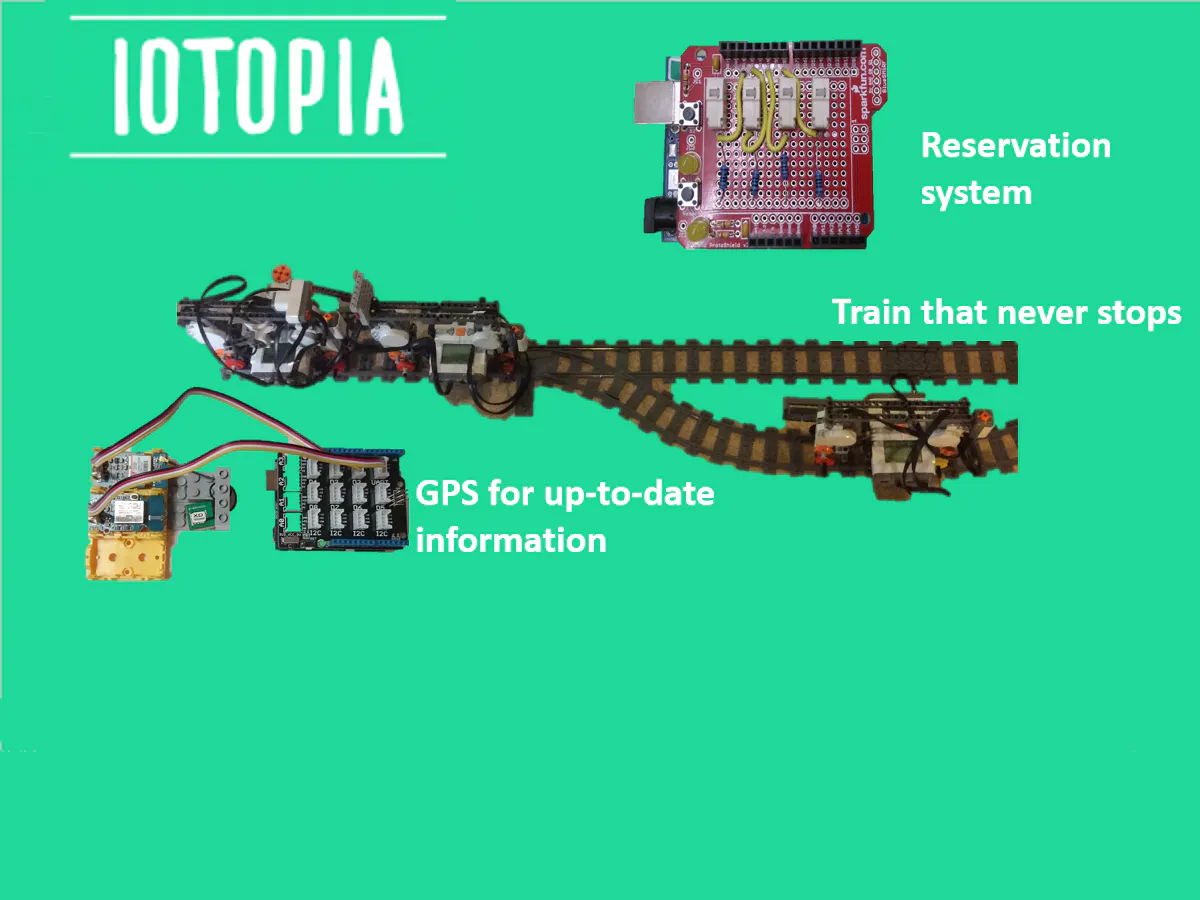## Things used in this project

### Hardware components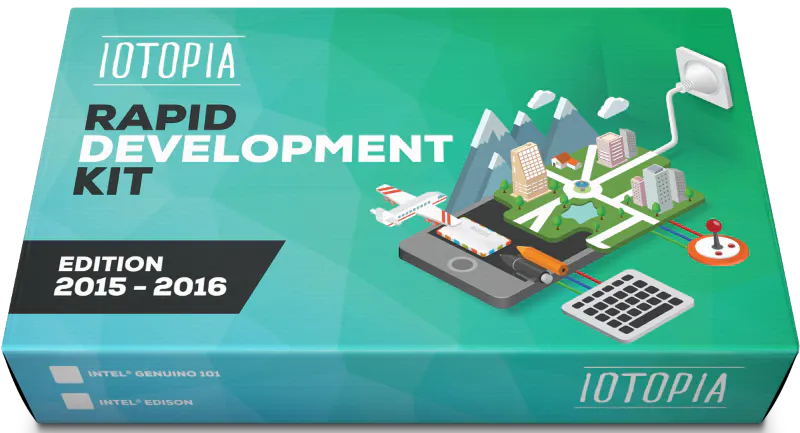AllThingsTalk IOTOPIA Rapid Development kit
×1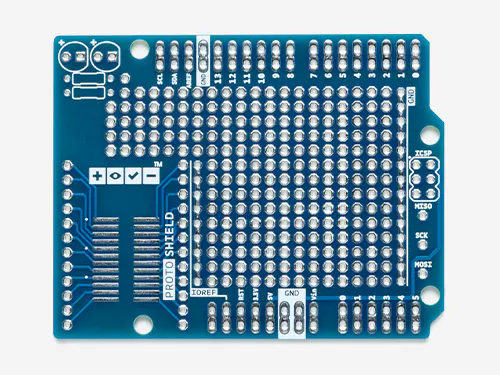Arduino Proto Shield
×1
 LEGO NXT robot
×1
 LEGO You can see the bricks above.
×1
 push buttons
×1SparkFun Pushbutton switch 12mm
×1Jumper wires (generic)
×1
 Lego rails
×1

### Hand tools and fabrication machines3D Printer (generic)

Solid Edge file

Solid Edge file

## Schematics

### GPS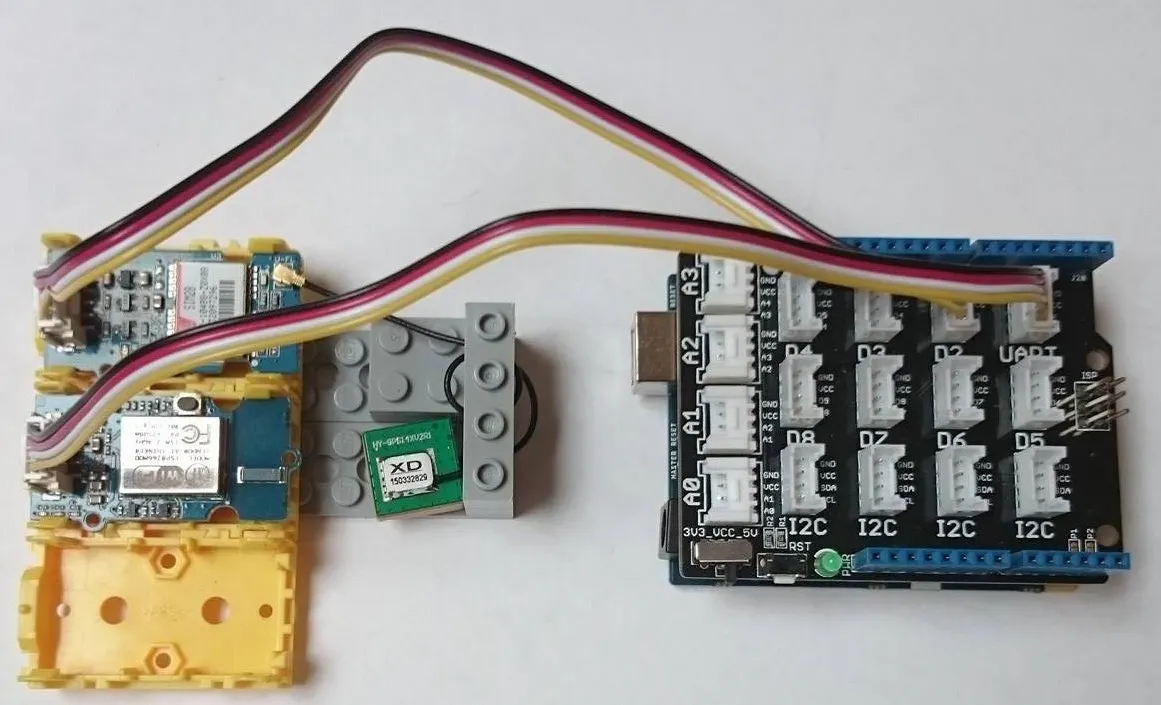### Push buttons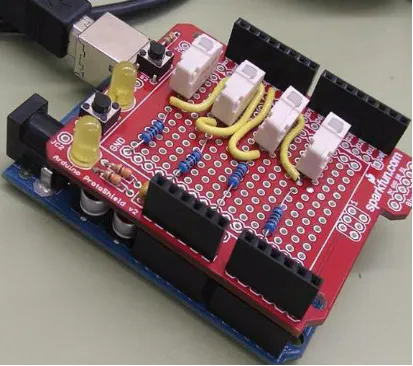## Code

### GPS code

Arduino
```#include "ATT_IOT_UART.h"      // AllThingsTalk Arduino UART IoT library
#include <SPI.h>               // Required to have support for signed/unsigned long type
#include "keys.h"              // Keep all your personal account information in a seperate file
#include <SoftwareSerial.h>

ATTDevice Device(&Serial1);
char httpServer[] = "api.smartliving.io";          // HTTP API Server host
char mqttServer[] = "broker.smartliving.io";       // MQTT Server Address

// Define PIN numbers for assets
// For digital and analog sensors, we recommend to use the physical pin id as the asset id
// For other sensors (I2C and UART), you can select any unique number as the asset id
#define gpsId 2                // Digital sensor is connected to pin D2 on grove shield

SoftwareSerial SoftSerial(2, 3);
unsigned char buffer;                   // Buffer array for data receive over serial port
int count=0;
int time= 30;                               // set time between reading coordinates (in seconds)

// Required for the device
void callback(int pin, String& value);

void setup()
{
Serial.begin(57600);                                 // Init serial link for debugging
while(!Serial && millis() < 1000);                   // Make sure you see all output on the monitor. After 1 sec, it will skip this step, so that the board can also work without being connected to a pc
Serial.println("Starting sketch");
Serial1.begin(115200);                               // Init serial link for WiFi module
while(!Serial1);

while(!Device.StartWifi())
Serial.println("Retrying...");
while(!Device.Init(DEVICEID, CLIENTID, CLIENTKEY))   // If we can't succeed to initialize and set the device credentials, there is no point to continue
Serial.println("Retrying...");
while(!Device.Connect(httpServer))                   // Connect the device with the AllThingsTalk IOT developer cloud. No point to continue if we can't succeed at this
Serial.println("Retrying");

Device.AddAsset(gpsId, "GPS module", "GPS", false, "{\"type\": \"object\",\"properties\": {\"lat\": {\"type\": \"number\", \"unit\": \"°\"},\"lng\": {\"type\": \"number\", \"unit\": \"°\"},\"alt\": {\"type\": \"number\", \"unit\": \"°\"},\"time\": {\"type\": \"integer\", \"unit\": \"epoc time\"}},\"required\": [\"lat\",\"lng\",\"alt\",\"time\" ]}");   // Create the sensor asset for your device

delay(1000);                                         // Give the WiFi some time to finish everything
while(!Device.Subscribe(mqttServer, callback))       // Make sure that we can receive message from the AllThingsTalk IOT developer cloud (MQTT). This stops the http connection
Serial.println("Retrying");

//pinMode(gpsId, INPUT);                               // Initialize the digital pin as an input.
SoftSerial.begin(9600);                 // the SoftSerial baud rate
Serial.println("GPS module is ready for use!");
delay(2000);
}

float longitude;
float latitude;
float altitude;
float timestamp;
float timestamp1;
float longitude1;
float latitude1;
float distance;
float time_left;
float station = 15.81;

void loop()
{
{
delay(time*1000);
}

Device.Process();
delay(5000);
}

void SendValue()
{
float distance = acos(sin(latitude*3.141592654/180)*sin(latitude1*3.141592654/180)+cos(latitude1*3.141592654/180)*cos(latitude*3.141592654/180)*cos(abs(longitude*3.141592654/180-longitude*3.141592654/180)))*6370*1000;
float Speed = distance/ time*3600;

Serial.println(sin(latitude*3.141592654/180)*sin(latitude1*3.141592654/180)+cos(latitude1*3.141592654/180)*cos(latitude*3.141592654/180)*cos(abs((longitude*3.141592654/180)-(longitude1*3.141592654/180))),20);

float time_left = station / Speed ;

Serial.print("sending gps data");
String data;
data = "{\"Distance\":" + String(distance, 10)+"km" + ",\"Speed\":" + String(Speed, 3)+"km/h" + ",\"Time left\":" + String(time_left, 3)+"h" + "}";
Device.Send(data, gpsId);
}

//////////////////////////////////////////////////////////////////////////////////////////////////////////////////////////////////////////////////////////////////////////////////////////////

// Callback function: handles messages that were sent from the iot platform to this device.
void callback(int pin, String& value)
{
Serial.print("incoming data for: ");         // Display the value that arrived from the AllThingsTalk IOT developer cloud.
Serial.print(pin);
Serial.print(", value: ");
Serial.println(value);
}

{
bool foundGPGGA = false;                          // Sensor can return multiple types of data, need to capture lines that start with \$GPGGA
if (SoftSerial.available())                     // If date is coming from software serial port ==> data is coming from SoftSerial shield
{
while(SoftSerial.available())               // Reading data into char array
{
buffer[count++]=SoftSerial.read();      // Writing data into array
if(count == 64)break;
}

foundGPGGA = count > 60 && ExtractValues();  // If we have less then 60 characters, then we have bogus input, so don't try to parse it or process the values
clearBufferArray();                          // Call clearBufferArray function to clear the stored data from the array
count = 0;
}
return foundGPGGA;
}

bool ExtractValues()
{
unsigned char start = count;
while(buffer[start] != '\$')        // Find the start of the GPS data -> multiple \$GPGGA can appear in 1 line, if so, need to take the last one.
{
if(start == 0) break;            // It's an unsigned char, so we can't check on <= 0
start--;
}
start++;                           // Remove the '\$', don't need to compare with that.
if(start + 4 < 64 && buffer[start] == 'G' && buffer[start+1] == 'P' && buffer[start+2] == 'G' && buffer[start+3] == 'G' && buffer[start+4] == 'A')      //we found the correct line, so extract the values.
{
start+=6;
timestamp = ExtractValue(start);
latitude = ConvertDegrees(ExtractValue(start) / 100);
start = Skip(start);
longitude = ConvertDegrees(ExtractValue(start)  / 100);
start = Skip(start);
start = Skip(start);
start = Skip(start);
start = Skip(start);
altitude = ExtractValue(start);
return true;
}
else
return false;
}

float ExtractValue(unsigned char& start)
{
unsigned char end = start + 1;
while(end < count && buffer[end] != ',')      // Find the start of the GPS data -> multiple \$GPGGA can appear in 1 line, if so, need to take the last one.
end++;
buffer[end] = 0;                              // End the string, so we can create a string object from the sub string -> easy to convert to float.
float result = 0.0;
if(end != start + 1)                          // If we only found a ',', then there is no value.
result = String((const char*)(buffer + start)).toFloat();
start = end + 1;
return result;
}

//////////////////////////////////////////////////////////////////////////////////////////////////////////////////////////////////////////////////////////////////////////////////////////////////////////////////////

{
bool foundGPGGA1 = false;                          // Sensor can return multiple types of data, need to capture lines that start with \$GPGGA
if (SoftSerial.available())                     // If date is coming from software serial port ==> data is coming from SoftSerial shield
{
while(SoftSerial.available())               // Reading data into char array
{
buffer[count++]=SoftSerial.read();      // Writing data into array
if(count == 64)break;
}

foundGPGGA1 = count > 60 && ExtractValues1();  // If we have less then 60 characters, then we have bogus input, so don't try to parse it or process the values
clearBufferArray();                          // Call clearBufferArray function to clear the stored data from the array
count = 0;
}
return foundGPGGA1;
}

bool ExtractValues1()
{
unsigned char start = count;
while(buffer[start] != '\$')        // Find the start of the GPS data -> multiple \$GPGGA can appear in 1 line, if so, need to take the last one.
{
if(start == 0) break;            // It's an unsigned char, so we can't check on <= 0
start--;
}
start++;                           // Remove the '\$', don't need to compare with that.
if(start + 4 < 64 && buffer[start] == 'G' && buffer[start+1] == 'P' && buffer[start+2] == 'G' && buffer[start+3] == 'G' && buffer[start+4] == 'A')      //we found the correct line, so extract the values.
{
start+=6;
timestamp1 = ExtractValue(start);
latitude1 = ConvertDegrees(ExtractValue1(start) / 100);
start = Skip(start);
longitude1 = ConvertDegrees(ExtractValue1(start)  / 100);
start = Skip(start);
start = Skip(start);
start = Skip(start);
start = Skip(start);
altitude = ExtractValue1(start);
return true;
}
else
return false;
}

float ExtractValue1(unsigned char& start)
{
unsigned char end = start + 1;
while(end < count && buffer[end] != ',')      // Find the start of the GPS data -> multiple \$GPGGA can appear in 1 line, if so, need to take the last one.
end++;
buffer[end] = 0;                              // End the string, so we can create a string object from the sub string -> easy to convert to float.
float result = 0.0;
if(end != start + 1)                          // If we only found a ',', then there is no value.
result = String((const char*)(buffer + start)).toFloat();
start = end + 1;
return result;
}

//////////////////////////////////////////////////////////////////////////////////////////////////////////////////////////////////////////////////////////////////////////////////////////////////////////////
float ConvertDegrees(float input)
{
float fractional = input - (int)input;
return (int)input + (fractional / 60.0) * 100.0;
}

unsigned char Skip(unsigned char start)
{
unsigned char end = start + 1;
while(end < count && buffer[end] != ',')      // Find the start of the GPS data -> multiple \$GPGGA can appear in 1 line, if so, need to take the last one.
end++;
return end+1;
}

void clearBufferArray()                         // Function to clear buffer array
{
for (int i=0; i<count; i++)
{
buffer[i] = NULL;                           // Clear all index of array with command NULL
}
}
```

### GPS CODE - keys.h

Arduino
In order to see the the time left till the train comes. You have to make an account on smartliving and next fill in the deviceid, clientid, clientkey
```#ifndef SETTINGS
#define SETTINGS

#define DEVICEID "******"            // Your device id comes here
#define CLIENTID "*****"            // Your client id comes here;
#define CLIENTKEY "*****"           // Your client key comes here;

#endif
```

### Buttons

Arduino
```/*
Reads a digital input on pin 2, prints the result to the serial monitor

This example code is in the public domain.
*/

// digital pin 2 has a pushbutton attached to it. Give it a name:
int pushButton1 = 13;
int pushButton2 = 12;
int pushButton3 = 10;
int pushButton4 = 8;

// the setup routine runs once when you press reset:
void setup() {
// initialize serial communication at 9600 bits per second:
Serial.begin(9600);
// make the pushbutton's pin an input:
pinMode(pushButton1, INPUT);
pinMode(pushButton2, INPUT);
pinMode(pushButton3, INPUT);
pinMode(pushButton4, INPUT);
}

// the loop routine runs over and over again forever:
void loop() {
String char1;

if (buttonState1 == 1) Serial.print("A");
if (buttonState2 == 1) Serial.print("B");
if (buttonState3 == 1) Serial.print("C");
if (buttonState4 == 1) Serial.print("D");
delay(150);

}
```

## Credits

### Tom Legein

1 project • 3 followers

### Stijn Luchie

1 project • 3 followers

### Thijs Lescroart

1 project • 3 followers